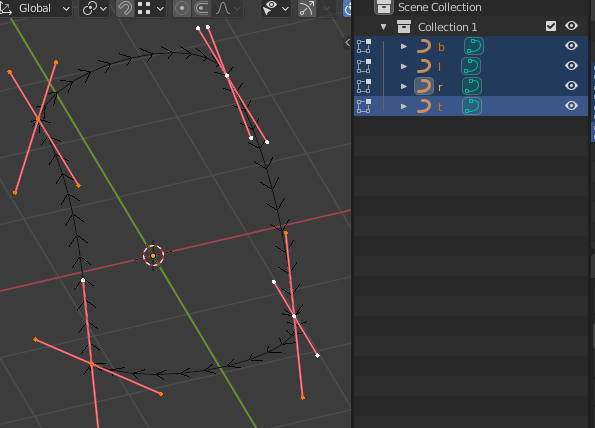# Sorting the curves?

Say for example there are these 4 curve objects. Each also is snapped onto a vertex so they can form a patch like this. Also you can see that I have manually and very carefully switched the direction of the curve so it flows in a clockwise order. Now consider doing this automatically.

I am interested to know if there is a recommended method or algorithm for sorting the curves that way. Ideally the top-left point should go first and the spline order can be clockwise.Forgive me if I am misreading your post, but it seems that what you wish to do is orchestrate an ordered sequence of curve objects such that the last control point of curve m is constrained in some fashion with respect to the first control point of curve m + 1. Perhaps the constraint is just positional: it suffices if the two points merely coincide and it does not matter if the join fails to be fair. Or perhaps the tangents must align, so that one end flows to the next without an obvious kink. Better yet, lets match up curvature across the join. Constraints vary, but the overarching construct is that of a Spline, an ordered sequence of piecewise elements constrained to join at their endpoints in accordance to characteristic rules. It seems to me that the end point coordination you are manually performing among the distinct curve objects t, r, b and l has its analog within the geometry data block of one curve object - but in this latter setting, Blender manages the mathematics for you - accepting some input from you on the end formulation of the constraint: perhaps setting the w parameter of a NURBS curve or specifying the types and positions of Bézier control handles. So what is the gain of replicating spline machinery among a sequence of curve objects when it already operates automagically at the geometry level of one curve object – or am I thoroughly misunderstanding your aims?

Thanks for the suggestions, I was able to follow some of your suggestions. I will try to roll with this one, I can continue tests with this to see if is actually useful.

``````class Line:
def __init__(self):
self.name = ''
self.point1 = [0,0]
self.point2 = [0,0]
def set_points(self, x1,y1 , x2,y2):
self.point1 = [x1,y1]
self.point2 = [x2,y2]
def __repr__(self):
return '{0}={1}:{2}'.format(self.name, self.point1, self.point2)

def createLines():
'''
0,0   1,0
|       |
0,1   1,1
'''

# simplifying the problem

# * assume coords are in xy rather than uv
#   (just to look nice on this view)

# * no 3d space used but 2d space

# * no curves used but lines
#   (I think I can exclude curve-related notions
#    and think in terms of points or edges)

# * assume that points are snapped onto each other
#   (no problem if they share the same coordinates
#   but most important is to think in terms of edges)

i = [Line(), Line(), Line(), Line()]
i.name = 'top'
i.set_points(0,0 , 1,0) #lr

i.name = 'right'
i.set_points(1,0 , 1,1) #tb

i.name = 'bottom'
i.set_points(1,1 , 0,1) #rl

i.name = 'left'
i.set_points(0,1 , 0,0) #bt

return i

def tryShuffle(lines):
# shuffle line order
import random
random.shuffle(lines)
# shuffle point directions by chance
for l in lines:
if random.random() >= 0.5:
print('shuffling points for', l.name)
t = l.point1
l.point1 = l.point2
l.point2 = t

def trySort(first:str, lines):
import math

# somehow i found the first line to be this
# (later will find some better way to determine this)
first = [l for l in lines if l.name == first]
first = first if len(first) == 1 else None
print('first line:', first.name)

dist = lambda p1, p2: math.sqrt((p2-p1)**2 + (p2 - p1)**2)
sortedlines = []

current = first
for loop in range(4): # it will have to run only 4 times
print('current', current.name)
sortedlines.append(current)

for other in lines:
# skip same
if current == other: continue

# point2 of current - should always match point1 of other
# (valid sequence goes like in clockwise order: point2-point1-point2-point1-...)
if dist(current.point2, other.point1) < 0.0001:
print('matched point1 of', other.name)
# this is a valid match because it respects the order
# switch current and repeat outer loop
current = other
break

# point2 of current - matches point2 of other
# (it means that other direction is flipped - better to flip the points here)
if dist(current.point2, other.point2) < 0.0001:
print('matched point2 of', other.name, '(but in wrong direction)')
# I just do the flip here and let the sort to the next iteration
tmp = other.point1
other.point1 = other.point2
other.point2 = tmp
current = other
loop -= 1
break
return sortedlines

def test():
lines = createLines()
print('created lines')
print(lines)
print('')

tryShuffle(lines)
print('shuffled lines')
print(lines)
print('')

lines = trySort('top', lines)

print('****')
print('sorted lines are')
print(lines)

test()
``````